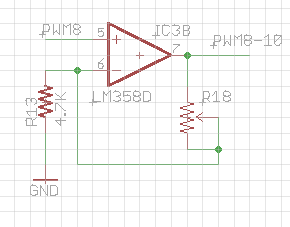# Using a 3.3v PWM signal to control a 5v PWM motor controller

I'd like to use a Teensy 3.2 (3.3v) PWM output to provide input to control a 15A 90v motor controller. With a 5v Arduino I get a great range of speed control, but looking at the motor controller (the original input is just a 100k pot across 0-5v) I think the input is just an R-C circuit to convert PWM to voltage. I'm thinking a 3.3v PWM signal will only give 3.3v max to that same circuit. Is there a simple voltage shifter circuit to boost the voltage from 3.3 to 5v? 5v is available from the motor controller. Motor Controller XY-C-1215

this is the circuit I use for my pwm controlleruse a 10k trimmer.

The trimmer can adjust the output between 3.3v and 10v.

adding more description for completeness. The circuit is a non inverting op-amp. the gain is 3.3v * (1+(R18/R13))

so if R18 timmer is 0 ohms, you get 3.3v output. at max, if R18 is 10k, your output is 3.3 (1+10/4.7) = 10.32v.

if you want fixed 5v output, use 2.4k for R18.

if you want signal to be analog, add an RC circuit to the output.

This should also work except the output would be inverted, am I correct? Only 3 tiny components would be a major plus.I think that will work.

If you want less component, you can setup the pin on the teensy to be open drain and just pullup to 5v.

I just found a simple motor controller that should do the trick using 2 PWM signals...

3.6A Brushed DC Motor Driver with Integrated Current Sensing (PWM Ctrl)

http://www.ti.com/product/drv8871?qgpn=drv8871

45v motor supply, bi-directional, selectable current limiting, logic levels are 3.3v or 5v tolerant. :)